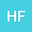Fundamentals of Relativization
•• Hontas Farmer
Hontas Farmer
Richard J. Daley College

Corresponding Author:[email protected]

Author Profile## Abstract

A new approach to reconciling General Relativity with Quantum Field Theory is Relativization, the act of making a physical model which obeys the principles of special relativity and General Relativity. This approach immediately yields results that no other approach has. I have established the foundations and fundamentals of relativization via a set of axioms. Expressions such as appear Pμ⁢|p>=kμ⁢|p>P^{\mu}\left|p\right\rangle\,=k^{\mu}\left|p\right\rangle, or ==kμ⁢=kμ\left\langle p\right|P^{\mu}\left|p\right\rangle\,=\,\left\langle p\right|k^{% \mu}\left|p\right\rangle\,=k^{\mu}\left\langle p\right.\left|p\right\rangle\,=% k^{\mu}. Such expressions appear in textbooks and papers but they are given a clearer interpretation in this model. Using this new approach to the problem, I will formulate the standard model as a relativized model in a curved space time with a locally valid graviton-Higgs interaction. This interaction will lead to a renormalized perturbation theory that can be summed up exactly to give the amplitude of graviton-graviton interaction as approximately C⁢o⁢s⁢h⁢(p)Cosh(p). I will solve a Schrodinger equation for a gravitationally bound system and get theoretical predictions relating to the thermodynamics of Planck scale black holes. Relativization has already provided a finite quantitative prediction for the quantum corrections to the local gravitational field, and gives results compatible with established black hole thermodynamics. Further research will certainly yield new insights.